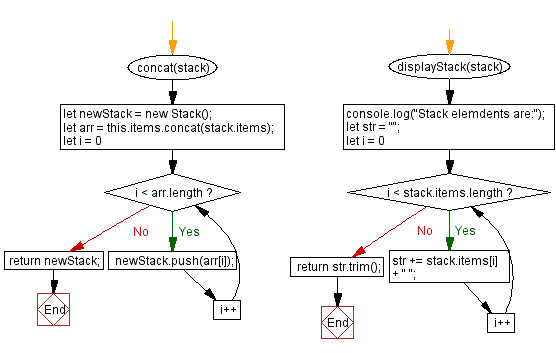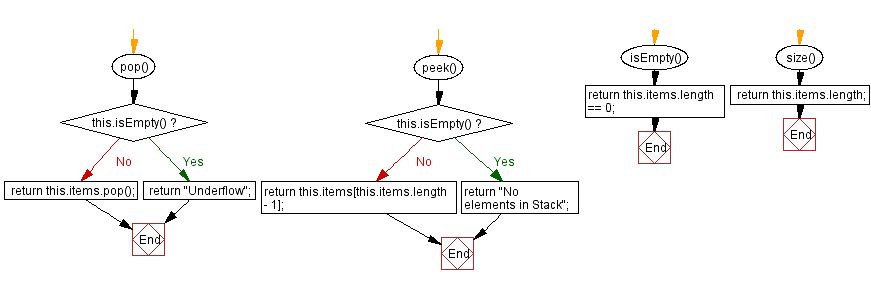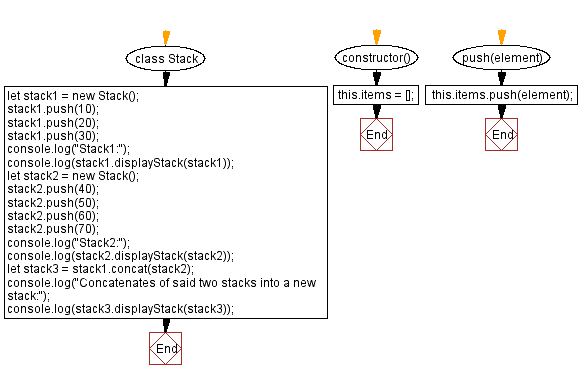# JavaScript Exercises: Concatenates two stacks into a new stack

## JavaScript Stack: Exercise-24 with Solution

Write a JavaScript program to implement a stack that supports concat() operation, which concatenates two stacks into a new stack.

Sample Solution:

JavaScript Code:

``````class Stack {
constructor() {
this.items = [];
}

push(element) {
this.items.push(element);
}

pop() {
if (this.isEmpty()) {
return "Underflow";
}
return this.items.pop();
}
peek() {
if (this.isEmpty()) {
return "No elements in Stack";
}
return this.items[this.items.length - 1];
}
isEmpty() {
return this.items.length == 0;
}
size() {
return this.items.length;
}
concat(stack) {
let newStack = new Stack();
let arr = this.items.concat(stack.items);
for (let i = 0; i < arr.length; i++) {
newStack.push(arr[i]);
}
return newStack;
}
displayStack(stack) {
console.log("Stack elements are:");
let str = "";
for (let i = 0; i < stack.items.length; i++)
str += stack.items[i] + " ";
return str.trim();
}
}
let stack1 = new Stack();
stack1.push(10);
stack1.push(20);
stack1.push(30);
console.log("Stack1:");
console.log(stack1.displayStack(stack1));
let stack2 = new Stack();
stack2.push(40);
stack2.push(50);
stack2.push(60);
stack2.push(70);
console.log("Stack2:");
console.log(stack2.displayStack(stack2));
let stack3 = stack1.concat(stack2);
console.log("Concatenates of said two stacks into a new stack:");
console.log(stack3.displayStack(stack3));
```
```

Sample Output:

```Stack1:
Stack elements are:
10 20 30
Stack2:
Stack elements are:
40 50 60 70
Concatenates of said two stacks into a new stack:
Stack elements are:
10 20 30 40 50 60 70
```

Flowchart:Live Demo:

See the Pen javascript-stack-exercise-24 by w3resource (@w3resource) on CodePen.

Improve this sample solution and post your code through Disqus

Stack Previous: Create stacks from arrays.
Stack Exercises Next: Create a copy of the stack.

What is the difficulty level of this exercise?

Test your Programming skills with w3resource's quiz.

﻿